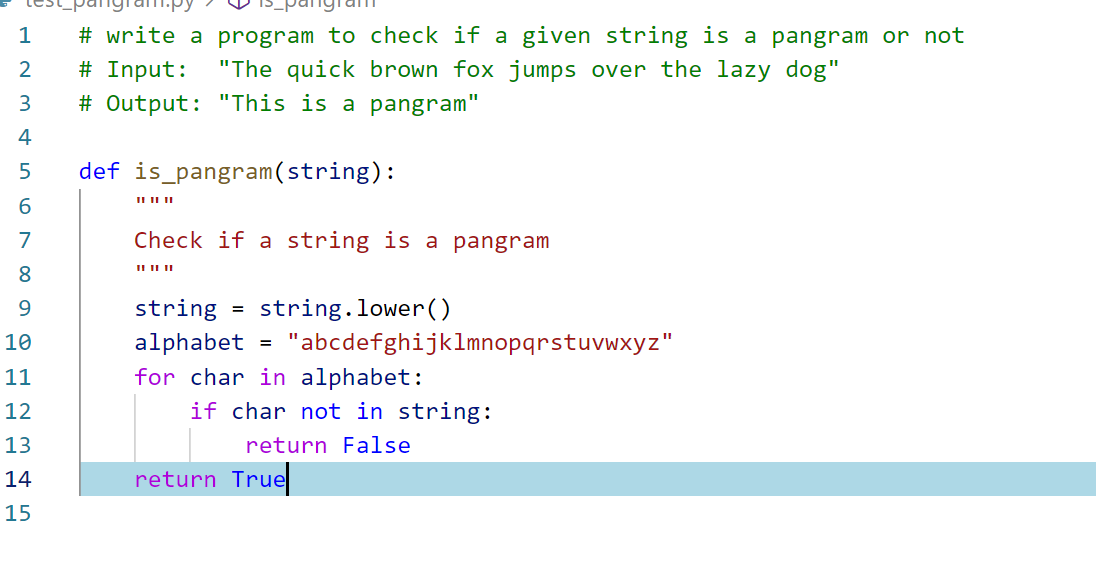Consider the case of buiding a movie recommendation. We usually use collabrative filtering technique to recommend a specific movie to a user of XYZ platform. Yet how will we categorize the movies into various categories? We can do a decent job to recommend movies based on category of movie it belongs to like blockbuster, comic etc. as shown in the picture: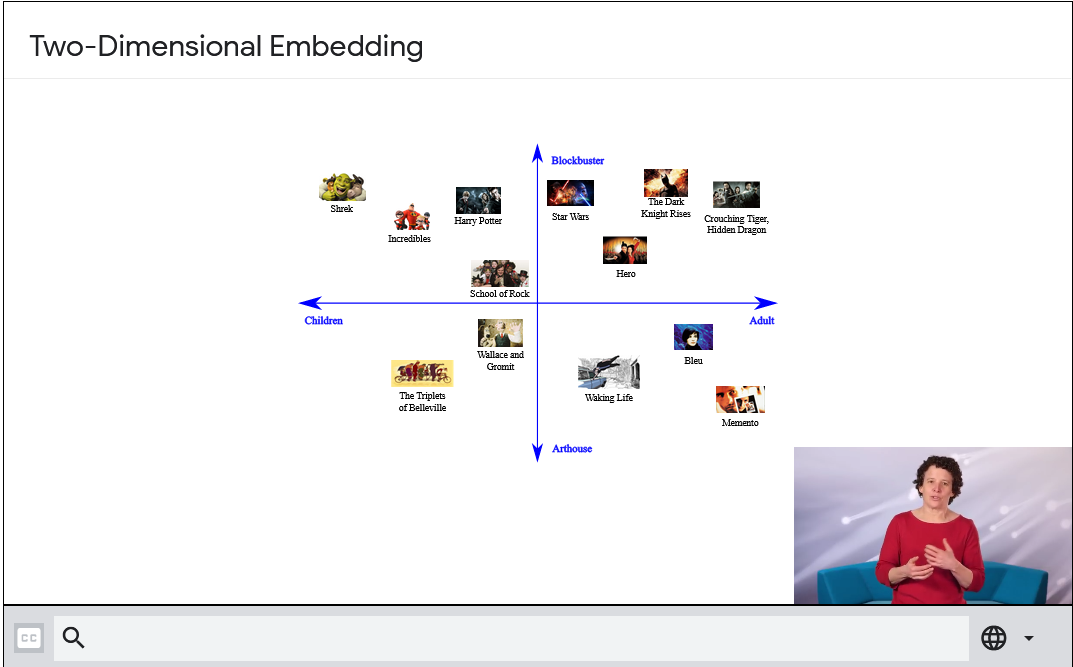Resource 1

Yet realisatically, we won’t be able to identify our users taste by just the categories, as people are more diverse in nature. So this is usually identified by understanding the latent factors corresponding to various users and movies. So in a scale of ratings of each movies we can map how much users like it by calculating latent factors as done with `pandas cross-tab` functionality. This is as shown in below diagram representing movies and users.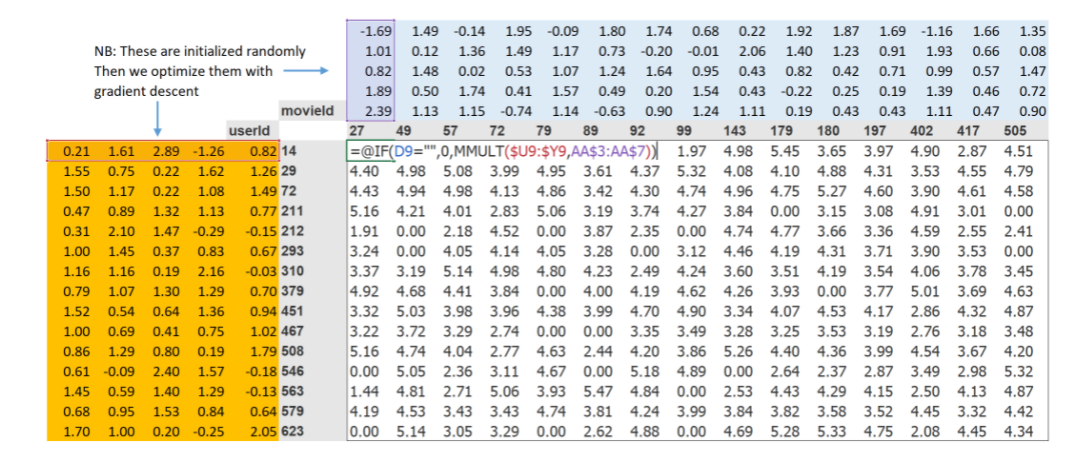Consider the case of a large orgs like netflix with millions of movies listed. Yet the no of movies watched by a user for our example, be corresponding to a very empty matrix who just watched 3 movies. We are going to predict, how much he will like our movies in full list and give some recommendations for the user.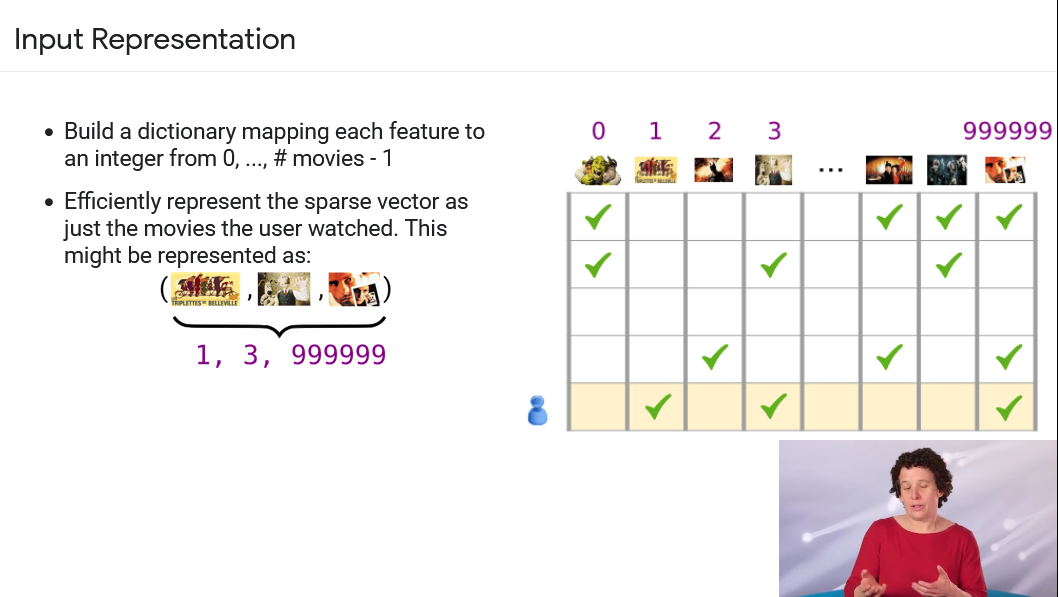So how are we going to calculate the remaining predictions for rest of movies. It’s a 3 step process as mentioned in Resource 2:

1. Randomly initialize some parameters
2. Calculate our predictions
3. Calculate our loss

In Deep Learning frameworks, the idea of pandas cross tab to represent the latent factors - which represent the distinct of a matrix is not present. To represent our movies like in the below diagram. We need to calculate our particular movie and user combination. Then identify index of our movie in `user latent factor matrix` and index in `movie latent factor matrix`. Then you need to multiply the predictions dot product.

The trick is to replace our indices with one-hot encoded vectors to multiply by user factors as shown in code, to calucate latent factors:

``````one_hot_3 = one_hot(3, n_users).float()
user_factors.t() & one_hot_3
``````

So in frameworks like Pytorch, multiplying by a one-hot encoded matrix using the computational shortcut that it can be implemented by simply indexing directly. This is quite a fancy word for a very simple concept. The thing that you can multiply the one hot-encoded matrix by (or using a computational shortcut index into directly) is called Embedding matrix.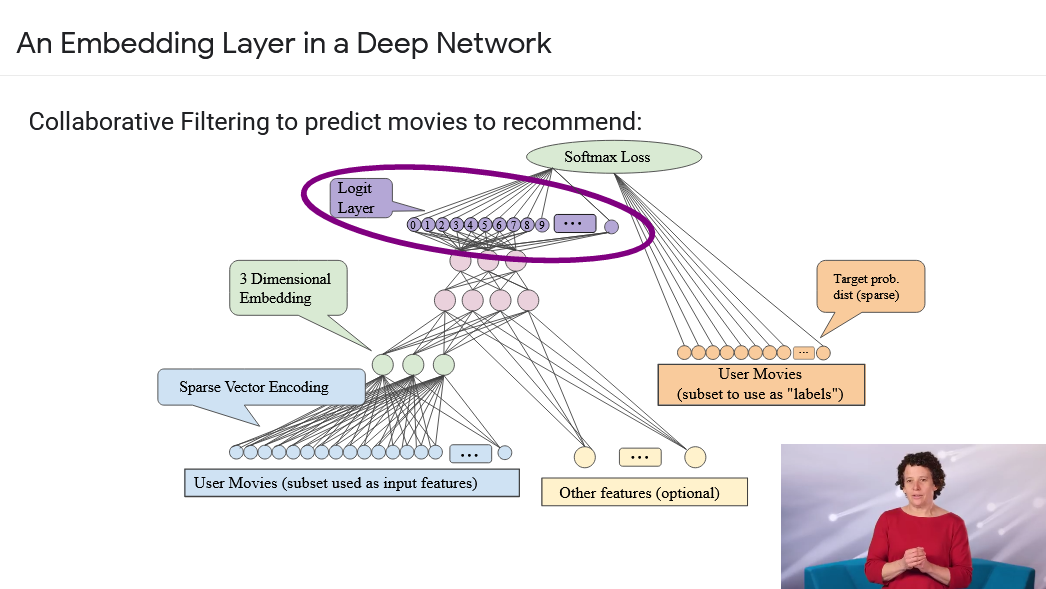Let’s look at a practical example of Embedding in real world using github copilot, which uses language modelling over Codex.